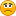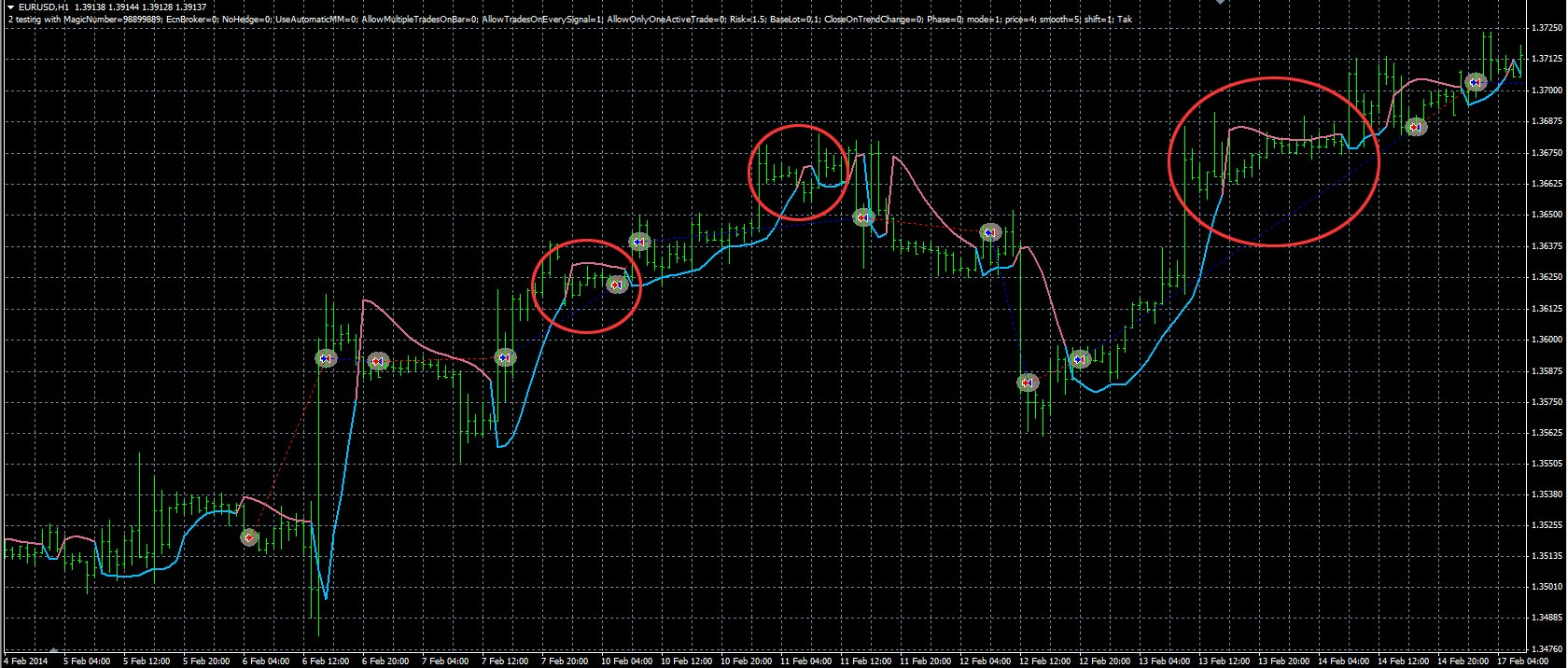# Requests & Ideas - page 317382

mrtools:
Gfuchs, would you prefer to not have the mtf Gann part, think that could be causing the problem your having? When I coded it was using 4 hour Adaptive Gann as major trend and taking trades when lesser time frame Gann changed to be the same as the 4 hour Gann. The volty trailing shouldn't be causing the problem especially if you have it deactivated.

Hi Mladen or mrtools, if the mtf Gann part is causing that error then yes I can live without it. Could you be so kind to remove that option then? Thanks a lot in advance!241
dannyla

Dear sir

Is it possible to build the ribbon version of this indicator.

Ribbon, filled with positive or negative color the crossing of two half moving averages.

Files:162720

dasssi:
Dear sir

Is it possible to build the ribbon version of this indicator.

Ribbon, filled with positive or negative color the crossing of two half moving averages.

dasssi,

This would be an averages ribbon (can combine any of the 20 types of averages into a ribbon) : averages_ribbon.mq4

The averages that can be combined into a ribbon :
0 = SMA

1 = EMA

2 = Double smoothed EMA

3 = Double EMA (DEMA)

4 = Triple EMA (TEMA)

5 = Smoothed MA

6 = Linear weighted MA

7 = Parabolic weighted MA

8 = Alexander MA

9 = Volume weighted MA

10 = Hull MA

11 = Triangular MA

12 = Sine weighted MA

13 = Linear regression

14 = IE/2

15 = NonLag MA

16 = Zero lag EMA

18 = Super smoother

19 = Smoother60

to the nmc one ? It's from this post:

https://www.mql5.com/en/forum/general162720

MikhaelPankov:

to the nmc one ? It's from this post:

https://www.mql5.com/en/forum/general

Mikhael

Files:60

Mladen, great job, as usual. Thank you!162720

Step ma of probability density function moving average (odf ma) made multi time frame : stepma_pdf_nmc_3.01_mtf.mq4

Files:9493

Gfuchs:
Hi Mladen or mrtools, if the mtf Gann part is causing that error then yes I can live without it. Could you be so kind to remove that option then? Thanks a lot in advance!

Gfuchs removed the mtf Gann part, please try this version.382

mrtools:
Gfuchs, would you prefer to not have the mtf Gann part, think that could be causing the problem your having? When I coded it was using 4 hour Adaptive Gann as major trend and taking trades when lesser time frame Gann changed to be the same as the 4 hour Gann. The volty trailing shouldn't be causing the problem especially if you have it deactivated.
mrtools:
Gfuchs removed the mtf Gann part, please try this version.

Still doesn't workThe signals are different...Files:241
dannyla
dasssi,

This would be an averages ribbon (can combine any of the 20 types of averages into a ribbon) : averages_ribbon.mq4

The averages that can be combined into a ribbon :
0 = SMA

1 = EMA

2 = Double smoothed EMA

3 = Double EMA (DEMA)

4 = Triple EMA (TEMA)

5 = Smoothed MA

6 = Linear weighted MA

7 = Parabolic weighted MA

8 = Alexander MA

9 = Volume weighted MA

10 = Hull MA

11 = Triangular MA

12 = Sine weighted MA

13 = Linear regression

14 = IE/2

15 = NonLag MA

16 = Zero lag EMA

18 = Super smoother

19 = Smoother

Dear sir

is it possible do the same indicator for MT5.

Including: